# How to Sort Column by Length of Cell Characters in Excel

This post will guide you how to sort column values by length of the characters in one column in Excel. How do I sort a list of column by character length in Excel 2013/2016.

## Sort Column by Length of Cell Characters using Sort Feature

Assuming that you have a list of data in column A which contain text string with different length. And you want to sort those data by character length in Column A. How do do it. This post will show you how to sort data by length of cell values.

To sort Column by length of Cell characters using Sort function, you still need to use a helper column to calculate the number of characters in Column A using LEN function, then use Sort option to sort those data. Do the following steps:

Step1: enter the following formula based on LEN function in the adjacent Column B to calculate the length of text string in Column A. Then drag the AutoFill Handle in Cell B1 down to other cells to apply this formula.

=LEN(A1)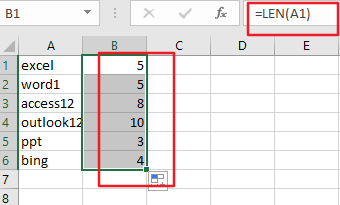Step2:  go to Data tab in the Excel Ribbon, and click Sort button under Sort & Filter group. And the sort Warning dialog will open.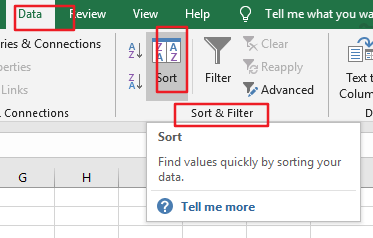Step3: select Expand the selection option in the Sort Warning dialog box. And click Sort button. Then the Sort dialog box will appear.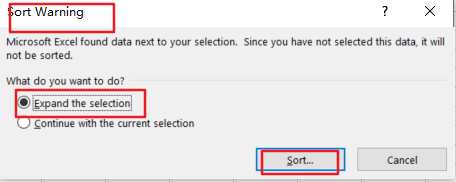Step4: select Column B in the dropdown list box of Sort by, and choose Cell Values in the drop down list box of Sort on, choose the sort order as Smallest to largest in the Order drop down list box. Click OK button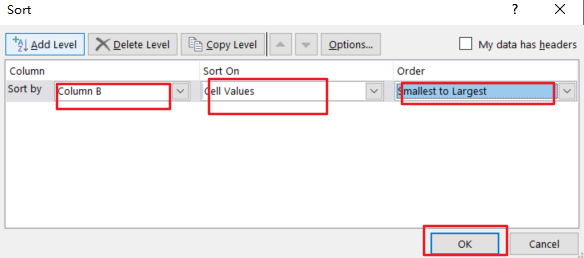Step5: you should see that column A has been sorted by the length of Cell values. Now you can delete the helper column.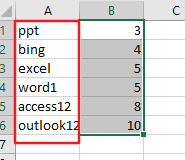### Related Functions

• Excel LEN function
The Excel LEN function returns the length of a text string (the number of characters in a text string).The syntax of the LEN function is as below:= LEN(text)…

### You might also like:

Sidebar